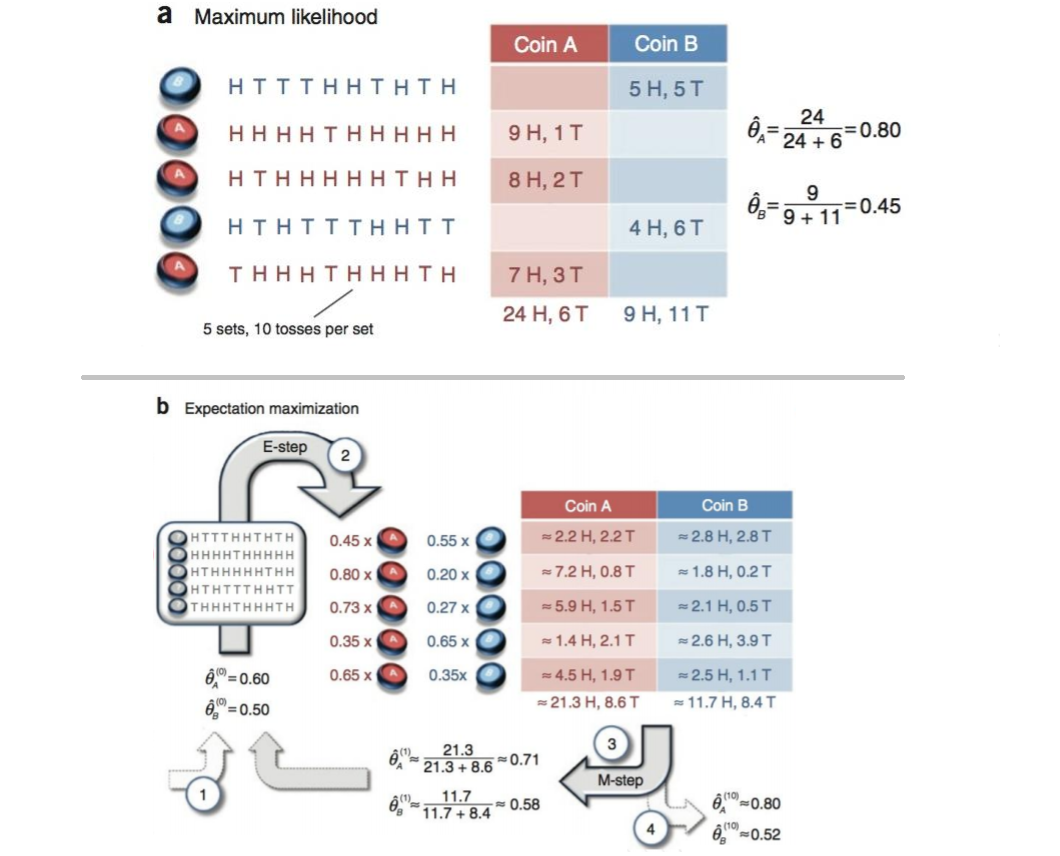EM算法

一、EM算法函数

1.1 EM算法问题导入

$~~~~~~~$调查我们学校的男生和女生的身高分布。 假设你在校园里随便找了100个男生和100个女生。调查学生的身高分布。将他们按照性别划分为两组，然后先统计抽样得到的100个男生的身高。假设他们的身高是服从正态分布的。但是这个分布的均值μ和方差${\sigma }^{2}$$σ^2$我们不知道，这两个参数就是我们要估计的。

$~~~~~~~$问题分析：设样本集$X={x}_{1},{x}_{2},\dots ,{x}_{N},$$X=x_1,x_2,…,x_N,$(N=100), $p\left({x}_{i}|\theta \right)$$p(x_i|θ)$为概率密度函数，表示抽到男生${x}_{i}$$x_i$的概率。

$~~~~~~~$找到一个参数θ，使得抽到X这组样本的概率最大，也就是说需要其对应的似然函数L(θ)最大。满足条件的θ叫做θ的最大似然估计量:

1.2 求解最大似然函数 估计值
1. 对 似然函数$L\left(\theta \right)$$L(θ)$ 取对数：
2. 对上式求导，令导数为 0 ，得到似然方程。
3. 求解似然方程，得到的参数 θ 即为所求。
1.3 符合高斯分布 概率密度函数

$~~~~~~~$若给定一组样本${x}_{1},{x}_{2}\dots {x}_{n}，$$x_1,x_2…x_n，$已知它们来自于高斯分布N(μ,σ)，试估计参数 μ,σ。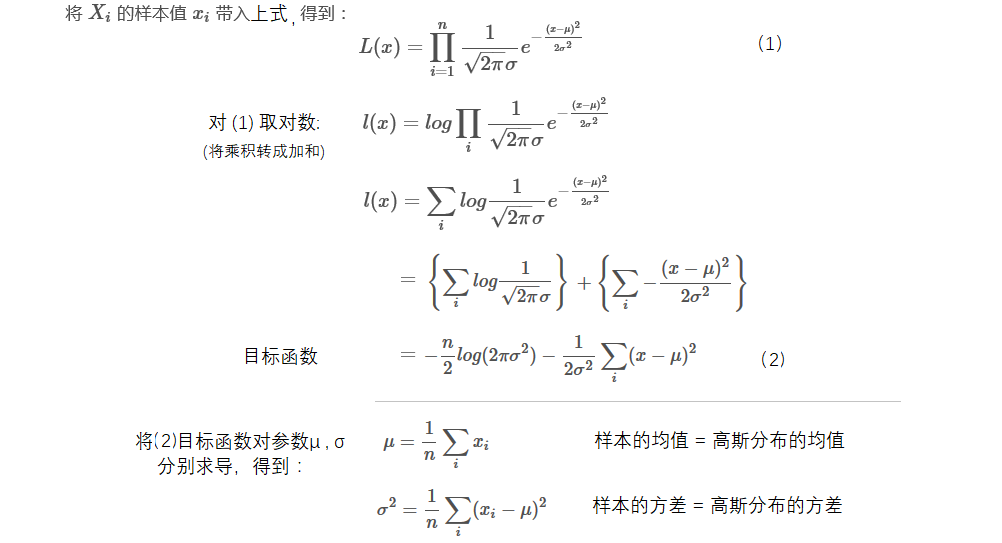1.3 混合高斯模型
直观理解猜测GMM的参数估计：
随机变量X是有K个高斯分布混合而成，取各个高斯分布的概率为π1π2... πK，
第i个高斯分布的均值为μi，方差为Σi。
若观测到随机变量X的一系列样本x1,x2,...,xn，试估计参数π，μ，Σ。

混合高斯模型：通过数据点的分布来反推公式中的参数
蒙特卡洛方法：通过落入特定形状数据点的概率来反推落入特定形状面积。


二、Jensen不等式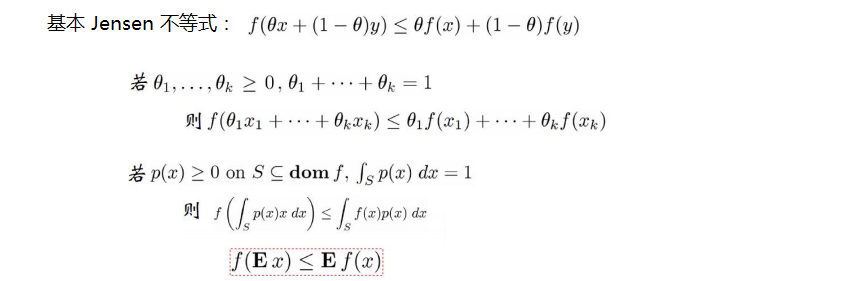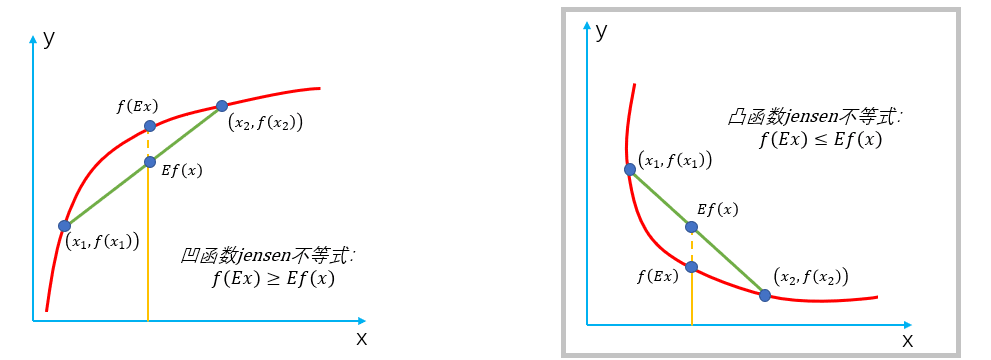2.1 lazy Statistician规则：

$~~~~~~~~~$设 y 是随机变量 x 的函数，$y=g\left(x\right)\left(g是连续函数\right)$$y = g(x)(g是连续函数)$，那么：

$~~~~~~~~~$(1) x 是离散随机变量，分布为$p\left(x={x}_{k}\right)={p}_{k},k\in {N}^{\ast },$$p(x = x_k) = p_k,k \in N^*,$$\sum_{k=1}^{\infty}~g(x)~p_k$绝对收敛，则：

$E\left(y\right)=E|g\left(x\right)|=\sum _{k=1}^{\mathrm{\infty }}g\left({x}_{k}\right){p}_{k}$

$~~~~~~~~~$(2) x 是连续随机变量，分布概率为$f\left(x\right)$$f(x)$,若${\int }_{-\mathrm{\infty }}^{\mathrm{\infty }}g\left(x\right)f\left(x\right)dx$$\int_{-\infty}^{\infty }g(x)f(x)dx$绝对收敛，则：

$E\left(y\right)=E|g\left(x\right)|={\int }_{-\mathrm{\infty }}^{\mathrm{\infty }}g\left(x\right)f\left(x\right)dx$

2.2 lazy Statistician 应用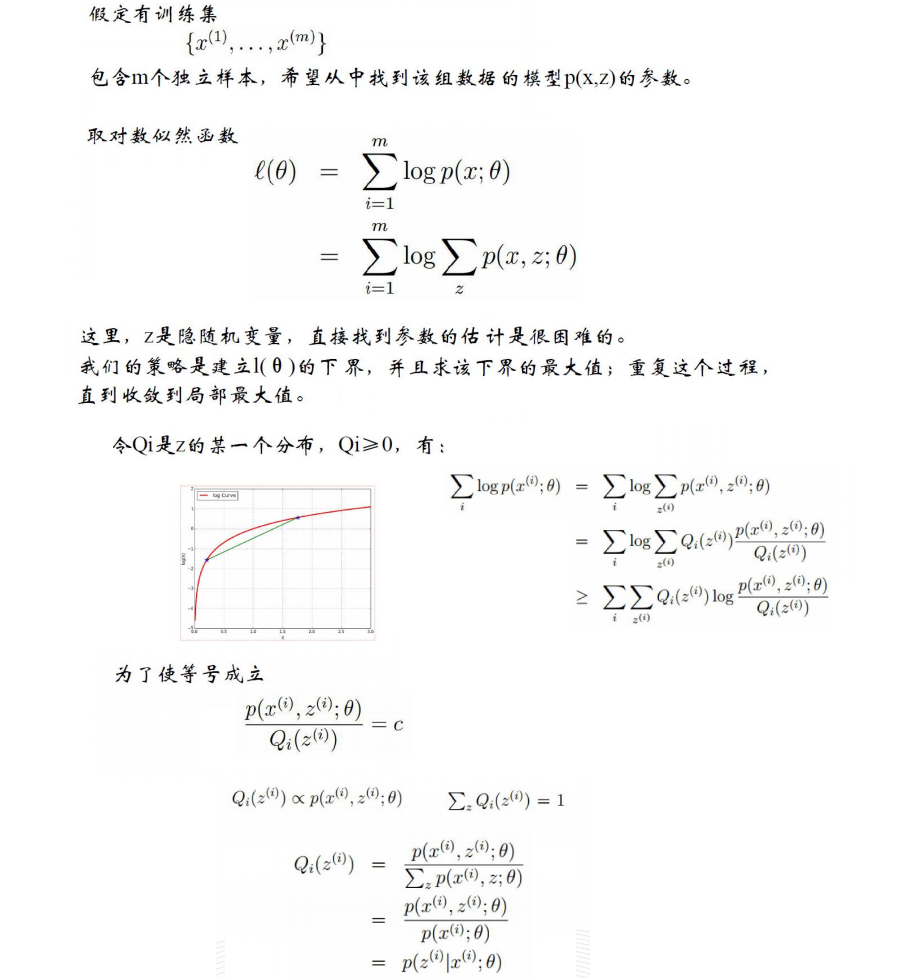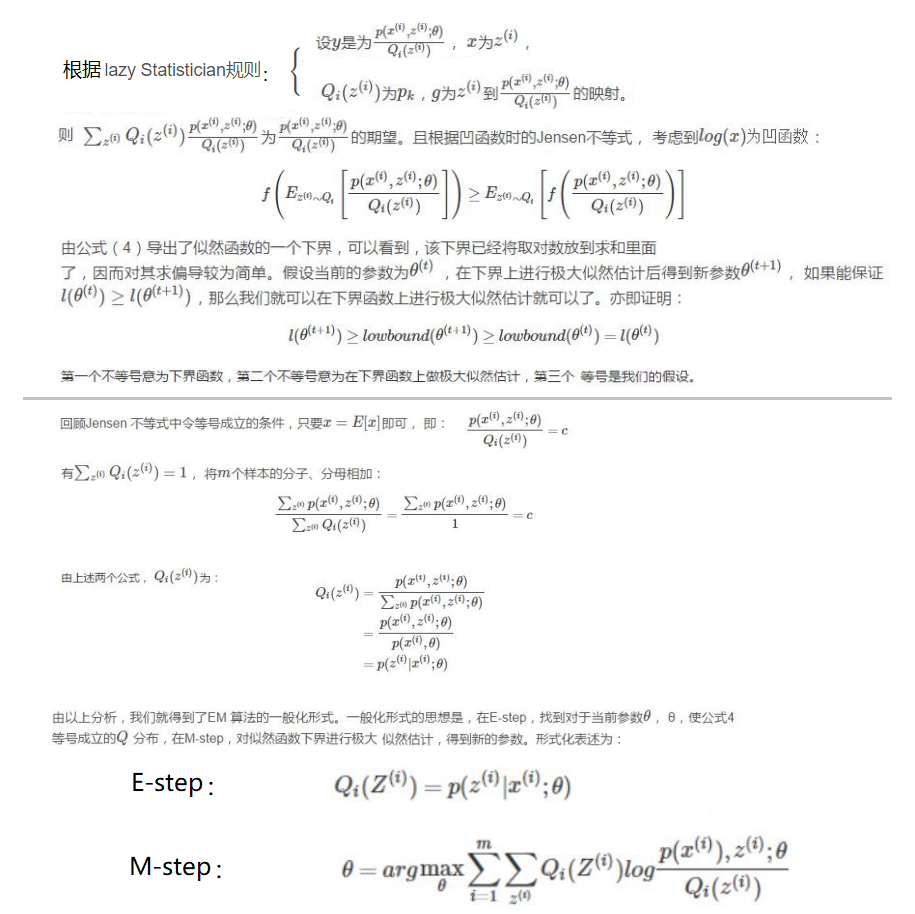三、算法流程

3.1 算法思路
直线式迭代优化的路径：
可以看到每一步都会向最优值前进一步，而且前进路线是平行于坐标轴的，因为每一步只优化一个变量。
这犹如在x-y坐标系中找一个曲线的极值，然而曲线函数不能直接求导，因此什么梯度下降方法就不适用了。

但固定一个变量后，另外一个可以通过求导得到，因此可以使用坐标上升法，一次固定一个变量，
对另外的求极值，最后逐步逼近极值。
对应到EM上， E步：固定θ，优化Q；
M步：固定Q，优化θ；
交替将极值推向最大。 （如图）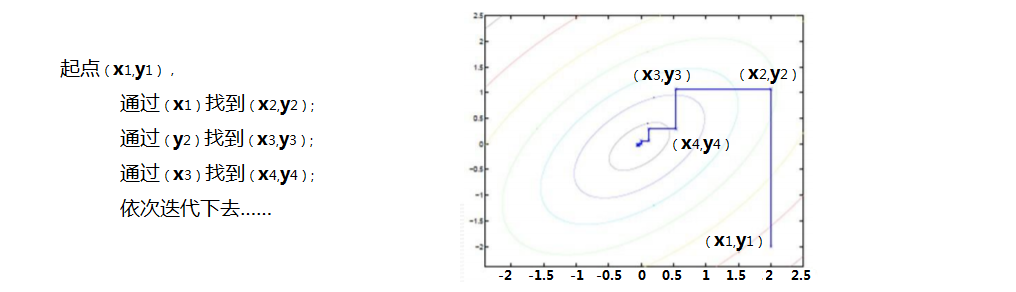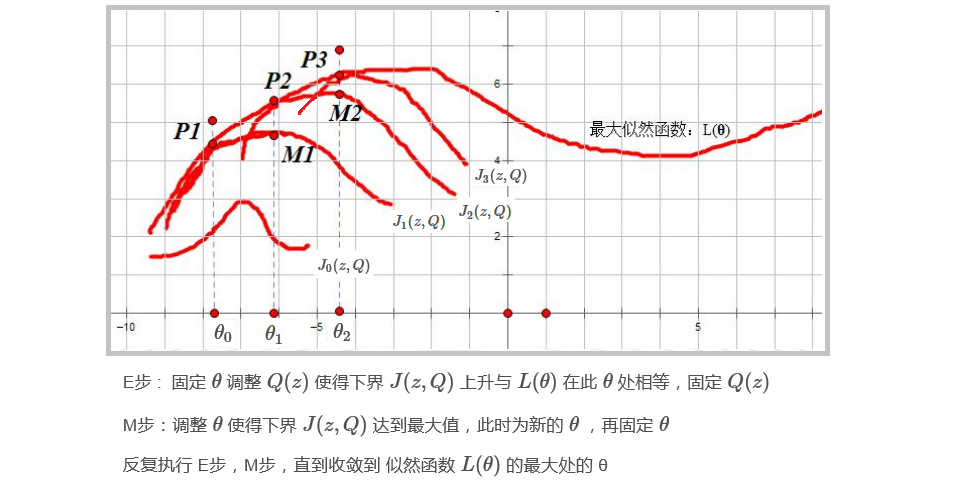3.2 EM 算法示例：

$~~~~~~~$● 假设现在有两枚硬币1和2,随机抛掷后正面朝上概率分别为${P}_{1}，{P}_{2}。$$P_1，P_2。$

 表一（实验数据） 表二（测试数据） 硬币 结果 统计 硬币 结果 统计 1 正正反正反 3正-2反 UnKnow 正正反正反 3正-2反 2 反反正正反 2正-3反 UnKnow 反反正正反 2正-3反 1 正反反反反 1正-4反 UnKnow 正反反反反 1正-4反 2 正反反正正 3正-2反 UnKnow 正反反正正 3正-2反 1 反正正反反 2正-3反 UnKnow 反正正反反 2正-3反

P1 = （3+1+2）/ 15 = 0.4
P2= （2+3）/10 = 0.5


$~~~~~~~$● 现在我们抹去每轮投掷时使用的硬币标记，如上右表（表二（测试数据））。

目标没变，还是估计P1和P2:
此时多了一个隐变量z，可以把它认为是一个5维的向量（z1,z2,z3,z4,z5)，
代表每次投掷时所使用的硬币，比如z1，就代表第一轮投掷时使用的硬币是1还是2。
但是，这个变量z不知道，就无法去估计P1和P2。



当假设P1 = 0.2，P2 = 0.8时：   （看看第一轮抛掷最可能是哪个硬币。）

如果是硬币1，得出3正2反的概率为：
0.2×0.2×0.2×0.8×0.8= 0.00512
如果是硬币2，得出3正2反的概率为：
0.8×0.8×0.8×0.2×0.2=0.03087
依次求出其他4轮中的相应概率。（如：表三）

 按照最大似然法则：（表三） 轮数 若是硬币1 若是硬币2 第1轮中最有可能的是硬币2 1 0.00512 0.03087 第2轮中最有可能的是硬币1 2 0.02048 0.01323 第3轮中最有可能的是硬币1 3 0.08192 0.00567 第4轮中最有可能的是硬币2 4 0.00512 0.02048 第5轮中最有可能的是硬币1 5 0.02048 0.01323
我们就把上面的值作为z的估计值。

P1 = （2+1+2）/15 = 0.33
P2=（3+3）/10 = 0.6

（下文中将这两个值称为P1和P2的真实值）。那么对比下我们初始化的P1和P2和新估计出的P1和P2：

 初始化P1 估计出P1 真实的P1 初始化P2 估计出P2 真实的P2 0.2 0.33 0.4 0.7 0.6 0.5
3.3 示例优化：
新估计出的 P1和P2 一定会更接近真实的 P1和P2

也就是说，我们使用了一个最可能的z值，而不是所有可能的z值。
所有的z值有多少个呢？显然，有2^5=32种，需要我们进行32次估值？？？


再次利用表三：我们可以算出每轮抛掷中使用硬币1或者使用硬币2的概率。
比如第1轮，使用硬币1的概率是：
0.00512/(0.00512+0.03087)=0.14
使用硬币2的概率是：
1-0.14=0.86

以P1估计为例，（表二）第1轮的3正2反相当于
0.14*3=0.42正
0.14*2=0.28反
依次算出其他四轮，表四如下：
new_P1=4.22/(4.22+7.98)=0.35


1 0.42 0.28
2 1.22 1.83
3 0.94 3.76
4 0.42 0.28
5 1.22 1.83

可以看到，改变了z值的估计方法后，新估计出的P1要更加接近0.4。原因就是我们使用了所有抛掷的数据，